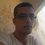# The limit of $(\sin n)$ does not exist.

Hello there,

Maybe the first thing that a calculus student when studying the sine and cosine function is that these are the first that he knows and do not have a limit as $x\to +\infty$.

But how about the sequence $(\sin n)$ and $(\cos n)$ ? do they have a limit ?

A method to prove that $(\sin n)$ does not have a limit is as follow :

• Assume that it has a limit then it should be a real number $l$ since $\sin$ is bounded.

• Since $\sin n\to l$, we get $\sin(2n) \to l$ and : $\cos(2n) \to 1-2l^2$.

• we have : $\sin 2 = \sin(2n+2-2n) =\sin(2n+2)\cos(2n) -\sin(2n)\cos(2n+2).$

But the limit of the right side is : $l(1-2l^2) -l(1-2l^2) =0.$

Which means that $\sin 2=0$ which is absurd.

If you liked it, share it. If you have question just write them.Note by Haroun Meghaichi
7 years, 2 months ago

This discussion board is a place to discuss our Daily Challenges and the math and science related to those challenges. Explanations are more than just a solution — they should explain the steps and thinking strategies that you used to obtain the solution. Comments should further the discussion of math and science.

When posting on Brilliant:

• Use the emojis to react to an explanation, whether you're congratulating a job well done , or just really confused .
• Ask specific questions about the challenge or the steps in somebody's explanation. Well-posed questions can add a lot to the discussion, but posting "I don't understand!" doesn't help anyone.
• Try to contribute something new to the discussion, whether it is an extension, generalization or other idea related to the challenge.
• Stay on topic — we're all here to learn more about math and science, not to hear about your favorite get-rich-quick scheme or current world events.

MarkdownAppears as
*italics* or _italics_ italics
**bold** or __bold__ bold
- bulleted- list
• bulleted
• list
1. numbered2. list
1. numbered
2. list
Note: you must add a full line of space before and after lists for them to show up correctly
paragraph 1paragraph 2

paragraph 1

paragraph 2

[example link](https://brilliant.org)example link
> This is a quote
This is a quote
    # I indented these lines
# 4 spaces, and now they show
# up as a code block.

print "hello world"
# I indented these lines
# 4 spaces, and now they show
# up as a code block.

print "hello world"
MathAppears as
Remember to wrap math in $$ ... $$ or $ ... $ to ensure proper formatting.
2 \times 3 $2 \times 3$
2^{34} $2^{34}$
a_{i-1} $a_{i-1}$
\frac{2}{3} $\frac{2}{3}$
\sqrt{2} $\sqrt{2}$
\sum_{i=1}^3 $\sum_{i=1}^3$
\sin \theta $\sin \theta$
\boxed{123} $\boxed{123}$

## Comments

Sort by:

Top Newest

Hi Haroun! Nice solution. One thing though, you are assuming that the limit of $sin(n)$ exists, for that the sequence $sin(n)$ needs to be monotonic and bounded. Obviously $sin(n)$ is bounded but what about monotonicity? It behaves differently( i.e. increasing/decreasing) in different intervals.

- 6 years, 11 months ago

Log in to reply

A convergent sequence does not have to be monotonic, for example $\frac{(-1)^n}{n}$.

If a sequence is bounded and monotonic then it converges (this an implication not an equivalence).

- 6 years, 11 months ago

Log in to reply

Obviously because there is not a set definition for the limit any oscillating series/function.

- 7 years, 2 months ago

Log in to reply

May I ask what math class you are in.

- 7 years, 2 months ago

Log in to reply

Haha, I'm in Algebra 1. I'm SUPPOSED to be in pre-calc or above, but my school-system is stupid. :D

- 7 years, 2 months ago

Log in to reply

In any interval of the form $[k\pi +\frac{\pi}{3},k\pi +\frac{2\pi}{3}]$, where $k$ is any natural number, there is at least a natural number $n_{k}$. The reason is that any such interval has length $\pi/3$ that is greater than 1. Since those intervals are mutually disjoint then the sequence $\{n_{k}\}$ is a sub-sequence of the sequence $\{n\}$ and, obviously, $|\sin n_k|\geq \frac{\sqrt{3}}{2}$. In a similar way, considering the intervals of the form $[k\pi -\frac{\pi}{6},k\pi +\frac{\pi}{6}]$, we can construct another sub-sequence $\{m_k\},$ of the sequence $\{n\},$ such that $|\sin m_k|\leq \frac{1}{2}.$ Assume that $\lim_{n\to \infty}\sin{n}$ exists and is the number $l.$ Using both sub-sequences defined above, we obtain that $|l|\geq \frac{\sqrt{3}}{2}$ and $|l|\leq \frac{1}{2}$, and this is a contradiction.

- 3 years, 3 months ago

Log in to reply

How you got sin2n tends to l ??

- 7 years, 2 months ago

Log in to reply

If a sequence $a_n$ converge to some real $l$ then all of it subsequences converge to $l$ this follows from the limit definition.

- 7 years, 2 months ago

Log in to reply

but how are you taking sin2n = l because as you are taking cos2n = 1 -2* l^2. can't we take sin2n=2sin(n)cos(n). and hence sin2n= 2l(sqrt(1-l^2))?

- 7 years, 1 month ago

Log in to reply

A much easier way to see that $sin(n)$ can't possibly have a limit is to note that since $sin(x)$ doesn't have a limit and is continuous, it can't possibly be that the limit at integral values exists. (I'll leave it to the interested reader to prove this rigorously.)

- 6 years, 11 months ago

Log in to reply

×

Problem Loading...

Note Loading...

Set Loading...